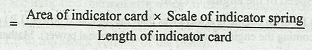# Indicated Mean Effective Pressure

The indicated mean effective pressure of an IC engine is obtained from the indicator diagram drawn with the help of an engine indicator. Mathematically, mean effective pressure (in bar)It may be noted that the mean effective pressure calculated on the basis of theoretical indicator diagram, is known as theoretical mean effective pressure. If it is based on the actual indicator diagram, then it is called actual mean effective pressure.